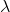# Sound Interference Problem -- Observer moving between two speakers

jybe

## Homework Statement

Two identical loudspeakers
are driven in phase by the same amplifier at a frequency of 680 Hz. The
speakers are 4.6 m apart. An observer stands 9 m away
from one of the speakers as shown. The observer
then starts moving directly towards the closest speaker.
How far does the observer have to move to hear
their first sound minimum? The speed of sound is 340 m/s

## The Attempt at a Solution

I found a solution:

Frequency of sound, f = 680 Hz
Velocity of sound, v = 340 m/s
Wavelength of sound,= v/f
= 340/680 = 0.5 m

Consider that after moving a distance 'd', the observer hear a minimum.
Distance to the first speaker, D1 = SQRT[(9 - d)2 + 4.62]
Distance to the second speaker, D2 = 9 - d
The condition of destructive interference is that,
D1 - D2 = n/2
SQRT[(9 - d)2 + 4.62] - (9 - d) = n/2
There are no solutions for n = 1 and n = 3, For n = 5,
SQRT[(9 - d)2 + 4.62] - (9 - d) = 1.25
SQRT[(9 - d)2 + 4.62] = 10.25 - d
Squaring both the sides,
[(9 - d)2 + 4.62] = [9.25 - d]2
81 + d2 - 18d + 21.16 = 105.0625 - 20.5d + d2
102.16 - 18d = 105.0625 - 20.5d
2.5d = 2.9025
d = 1.161 m

My question is about the bolded part. How was it determined that there are no solutions for n=1 and n=3? How do you know what n can equal? Thanks.

#### Attachments

Homework Helper
Gold Member
2022 Award
as shown
Please post either the diagram or a very clear description.

darth boozer
" An observer stands 9 m away
from one of the speakers as shown."

This is the image we need.

Homework Helper
Gold Member
Assuming the rest of work is correct, for ##n=1## you get the equation ##(9-d)^2+(4.62)^2=(9.25-d)^2## which has a solution with ##d## negative ##d=-33.56## I think. Similarly for ##n=3 ## you also get an equation with negative solution for ##d##. But ##d## must be positive, if we accept a negative ##d## as solution it would be like we are walking away from the source, instead of closer to the source.
So, ##n=5## is the first ##n## for which we get an equation with positive solution for ##d##.

Last edited: